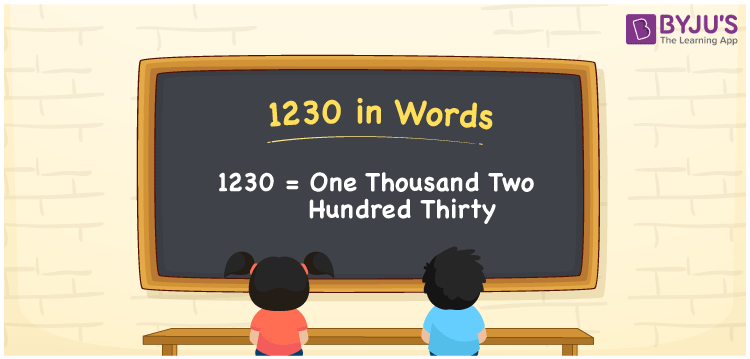# 1230 in Words

The number 1230 in words is one thousand two hundred thirty. In both the Indian and the International System of Numerals, the number 1230 is expressed as “One Thousand Two Hundred”. Hence, learning the place value system is essential for writing the number names. In this article, we are going to learn the spelling of 1230 in English with its procedure in detail.

 1230 in Words: One Thousand Two Hundred Thirty. One Thousand Two Hundred Thirty in Numerical Form: 1230.

## 1230 in English Words## How to Write 1230 in Words?

The following table will help you to understand the place values of the number 1230.

 Thousands Hundreds Tens Ones 1 2 3 0

The expanded form of 1230 is as follows:

= 1 × Thousand + 2 × Hundred + 3 × Ten + 0 × One

= 1 × 1000 + 2 × 100 + 3 × 10

= 1000 + 200 + 30

= 1230

= One thousand two hundred thirty

Hence, 1230 in words is one thousand two hundred thirty.

After 1229 but before 1231, the number 1230 appears.

1230 in words – One thousand two hundred thirty

Is 1230 an odd number? – No

Is 1230 an even number? – Yes

Is 1230 a perfect square number? – No

Is 1230 a perfect cube number? – No

Is 1230 a prime number? – No

Is 1230 a composite number? – Yes

## Frequently Asked Questions on 1230 in Words

Q1

### How to write 1230 in words?

1230 in words is one thousand two hundred thirty.

Q2

### Simplify 1200 + 30, and express it in words.

Simplifying 1200 + 30, we get 1230. Hence, 1230 in words is one thousand two hundred thirty.

Q3

### Is 1230 a perfect cube number?

No, 1230 is not a perfect cube number.

Test your Knowledge on 1230 in Words